# Square Root and Cube Root - Examples (with Solutions), Logical Reasoning Notes | Study Quantitative Aptitude (Quant) - CAT

## CAT: Square Root and Cube Root - Examples (with Solutions), Logical Reasoning Notes | Study Quantitative Aptitude (Quant) - CAT

The document Square Root and Cube Root - Examples (with Solutions), Logical Reasoning Notes | Study Quantitative Aptitude (Quant) - CAT is a part of the CAT Course Quantitative Aptitude (Quant).
All you need of CAT at this link: CAT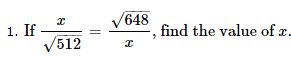A. 24

B. 12

C. 48

D. 36

Explanation :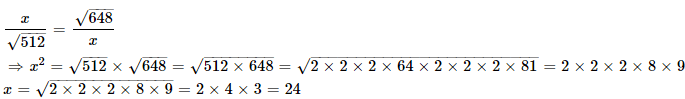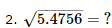A. 2.24

B. 1.24

C. 1.34

D. 2.34

Explanation :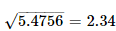3. If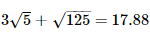, then what will be the value of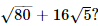A. 21.66

B. 13.41

C. 22.35

D. 44.7

Explanation :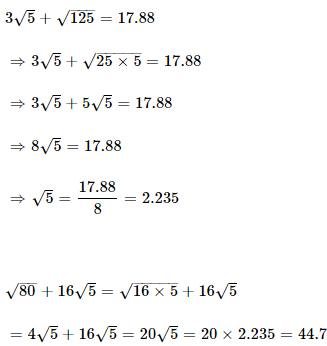4. The cube root of 0.000729 is

A. 0.09

B. 0.9

C. 0.21

D. 0.11

Explanation :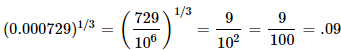5. What is the least perfect square which is divisible by each of 21, 36 and 66?

A. 213444

B. 214434

C. 214344

D. 231444

Explanation :

LCM of 21, 36, 66 = 2772
ie, all multiples of 2772 are divisible by 21, 36 and 66
Prime factorization of 2772 is,
2772 = 2 × 2 × 3 × 3 × 7 × 11
ie, to make it a perfect square, we have to multiply it by 7 and 11
Hence, required number = 2772 × 7 × 11 = 213444

The document Square Root and Cube Root - Examples (with Solutions), Logical Reasoning Notes | Study Quantitative Aptitude (Quant) - CAT is a part of the CAT Course Quantitative Aptitude (Quant).
All you need of CAT at this link: CATUse Code STAYHOME200 and get INR 200 additional OFF

## Quantitative Aptitude (Quant)

153 videos|129 docs|131 tests

### How to Prepare for CAT

Read our guide to prepare for CAT which is created by Toppers & the best Teachers

Track your progress, build streaks, highlight & save important lessons and more!

,

,

,

,

,

,

,

,

,

,

,

,

,

,

,

,

,

,

,

,

,

,

,

,

;